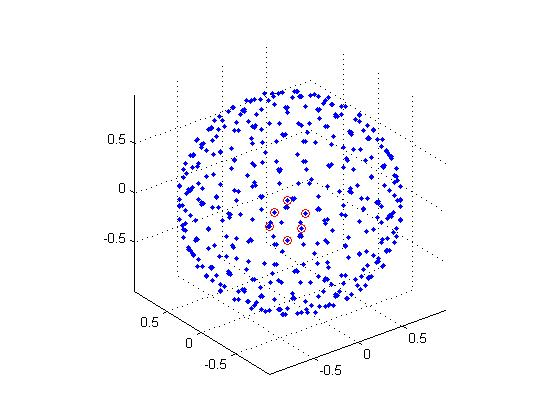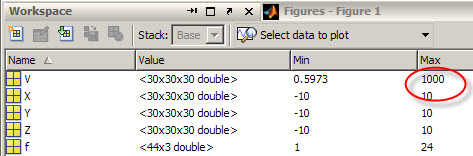# Unexpected is not wrong

I just worked on a case that typifies a situation I see in MATLAB fairly often. Unexpected results are not necessarily wrong results. I am a big fan of the “sanity check”, where if the results just seem “way out there” logically, something might be wrong.
• It could be the MATLAB code you wrote
• It could be the MATLAB code you got from someone else
• It could be your data
• It could be the MATLAB code we wrote
Being in technical support, I see a lot of the cases where MATLAB users think it is the last of these items. If it is, we own up to it and fix it. However, there is an army of people here at MathWorks running countless tests to ensure accuracy and stability of our code base. It is exceedingly rare for incorrect algorithms to sneak into the code. Today’s case was one where a MATLAB user doing an isosurface over a volume of data where the volume represented the distance from the origin. Any isosurfaces will simply be spheres. This makes sense. What did not make sense was there was a second much smaller sphere inside the first sphere. Here is a plot of all the vertices of the isosurface. Notice the points in red which form a secondary inner sphere.This was distressing, I looked through the code for a long time until I noticed in the workspace browser:It turns out that there was one data point in the middle of the sphere with a very high value. So, due to the interpolation that MATLAB is supposed to do for isosurface, there must be a sphere in the inside too because there will be a sharp gradient from this high outlier to the low values surrounding it.

MATLAB was doing exactly what it was supposed to do.

I never found out if that outlier was important. The right answer might be to NaN out that outlier and carry on with the expected results, or it that ‘extra’ isosurface might point to something important that would have been otherwise missed.

Have you had MATLAB give unexpected results, only to find out that there was something unexpected in your data?

|